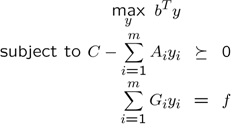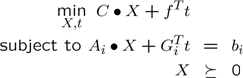# Automatic dualization

Tags:

Updated:

This tutorial is highly related to the paper Löfberg 2009 (which should be referenced if you use the functionality)

Important to know is that problems in YALMIP are modeled internally in the dual format (your problem is interpreted as a parametrization of the standard dual form). This means YALMIP by default matches your model description to the following form.In control theory and many other fields, this is the natural representation, but in some fields (e.g. combinatorial optimization), the primal form with an unstructured symmetric cone $$X$$ is often more natural.Due to the choice to work in the dual form, some problems are treated very inefficiently in YALMIP. Consider the following problem in YALMIP.

X = sdpvar(30,30);
Y = sdpvar(3,3);
obj = trace(X)+trace(Y);
F = [X>=0, Y>=0];
F = [F, X(1,3)==9, Y(1,1)==X(2,2), sum(sum(X))+sum(sum(Y)) == 20]
+++++++++++++++++++++++++++++++++++++++++++++++++++
|   ID|      Constraint|                      Type|
+++++++++++++++++++++++++++++++++++++++++++++++++++
|   #1|   Numeric value|   Matrix inequality 30x30|
|   #2|   Numeric value|     Matrix inequality 3x3|
|   #3|   Numeric value|   Equality constraint 1x1|
|   #4|   Numeric value|   Equality constraint 1x1|
|   #5|   Numeric value|   Equality constraint 1x1|
+++++++++++++++++++++++++++++++++++++++++++++++++++


Although the model looks like a problem in standard primal form, YALMIP will fit it to a dual form problem, since this is the standard model for YALMIP. YALMIP will explicitly parametrize the variable $$X$$ with 465 variables, $$Y$$ with 6 variables, create two semidefinite constraints and introduce 3 equality constraints in the dual form representation, corresponding to 471 equality constraint, 2 semidefinite cones and 3 free variables in the primal form. If we instead would have interpreted this directly in the stated primal form, we have 3 equality constraints, 2 semidefinite cones and no free variables, corresponding to a dual form with 3 variables and two semidefinite constraints. The computational effort is mainly affected by the number of variables in the dual form (equalities in primal) and the size of the semidefinite cones. Moreover, many solvers have robustness problems with free variables in the primal form (equalities in the dual form). Hence, in this case, this problem can probably be solved much more efficiently if we could use an alternative model.

The command dualize can be used to extract the primal form, and return the dual of this problem in YALMIPs preferred dual form (we never use this command in practice, see below where we show how to use an option in sdpsettings instead)

[Fd,objd,primals] = dualize(F,obj);Fd
+++++++++++++++++++++++++++++++++++++++++++++++++++
|   ID|      Constraint|                      Type|
+++++++++++++++++++++++++++++++++++++++++++++++++++
|   #1|   Numeric value|   Matrix inequality 30x30|
|   #2|   Numeric value|     Matrix inequality 3x3|
+++++++++++++++++++++++++++++++++++++++++++++++++++


If we solve this problem in dual form, the duals to the SDP constraints in Fd will correspond to the original variables $$X$$ and $$Y$$. The optimal values of these variables can be reconstructed easily (note that the dual problem is a maximization problem)

optimize(Fd,-objd);
for i = 1:length(primals);assign(primals{i},dual(Fd(i)));end


Variables are actually automatically updated, so the second line in the code above is not needed (but can be useful to understand what is happening). Hence, the following code is equivalent.

optimize(Fd,-objd);


The procedure can be applied also to problems with free variables in the primal form, corresponding to equality constraints in the dual form.

X = sdpvar(2,2);
t = sdpvar(2,1);
Y = sdpvar(3,3);
obj = trace(X)+trace(Y)+5*sum(t);

F = [sum(X) == 6+pi*t(1), diag(Y) == -2+exp(1)*t(2)]
F = [F, Y>=0, X>=0];

optimize(F,obj);
value(t)
ans =
-1.9099
0.7358
[Fd,objd,primals,free] = dualize(F,obj);Fd
+++++++++++++++++++++++++++++++++++++++++++++++++++
|   ID|      Constraint|                      Type|
+++++++++++++++++++++++++++++++++++++++++++++++++++
|   #1|   Numeric value|     Matrix inequality 3x3|
|   #2|   Numeric value|     Matrix inequality 2x2|
|   #3|   Numeric value|   Equality constraint 2x1|
+++++++++++++++++++++++++++++++++++++++++++++++++++


The detected free variables are returned as the 4th output, and can be recovered from the dual to the equality constraints (this is also done automatically by YALMIP in practice, see above).

optimize(Fd,-objd);
assign(free,dual(Fd(end)))
value(t)
ans =
-1.9099
0.7358


To simplify things even further, you can tell YALMIP to dualize, solve the dual, and recover the primal variables, by using the associated option.

optimize(F,obj,sdpsettings('dualize',1));
value(t)
ans =
-1.9099
0.7358


### More general models

Mixed problems can be dualized also, i.e. problems involving constraints of both dual and primal form. Constraint in dual form $$S(y)\succeq 0$$ are automatically changed to $$S(y)-X=0, X \succeq 0$$, and the dualization algorithm is applied to this new problem. Note that problems involving dual form semidefinite constraints typically not gain from being dualized, unless the dual form constraints are few and small compared to the primal form constraints.

A problem involving translated cones $$X \succeq C$$ where $$C$$ is a symmetric constant is automatically converted to a problem in standard primal form, with no additional slacks variables. Hence, a lower bound on a variable will typically reduce the size of a dualized problem, since no free variables or slacks will be needed to model this cone. Practice has shown that simple bound constraints of the type $$x \geq L$$ where $$L$$ is a large negative number can lead to problems if one tries to perform the associated variable change in order to write it as a simple LP cone. Essentially, the dual cost will contain large numbers. A primal problem $$\textbf{min } c^Tx, Ax=b, x \geq-L$$ will be converted to $$\textbf{min } c^Tz, Az=b+AL, z\geq 0$$ with the dual $$\textbf{min } (b+AL)Ty, A^Ty\leq c$$. If you want to avoid detection of translated LP cones (and thus treat the involved variables as free variables), set the 4th argument in dualize.

Problems involving second order cone constraints can also be dualized. A constraint of the type

x = sdpvar(n,1);
F = [cone(x(2:end),x(1))];


is a second order constraint in standard primal form. If your cone constraint violates this form, slacks will be introduced, except for translated second order cones, just as in the semidefinite case. Note that you need a primal-dual solver that can solve second order cone constraints natively in order to recover the original variables (currently SEDUMI and SDPT3 for mixed semidefinite second order cone problems, or MOSEK for pure second order cone problems).

Your solver has to be able to return both primal and dual variables for the reconstruction of variables to work. All SDP solvers do this, except LMILAB.

Primal matrices ($$X$$ and $$Y$$ in the examples above) must be defined in one simple call in order to enable detection of the primal structure. In other words, a constraint [X>=0] where X is defined with the code x = sdpvar(10,1);X = [x(1) x(6);x(6) x(2)] will not be categorized as a primal matrix, but as matrix constraint in dual form with three free variables.

### Primalize

For completeness, a functionality called primalize is available. This function takes an optimization problem in dual form and returns a YALMIP model in primal form. Consider the following SDP with 3 free variables, 1 equality constraint, and 1 SDP constraint of dimension 2.

C = eye(2);
A1 = randn(2,2);A1 = A1*A1';
A2 = randn(2,2);A2 = A2*A2';
A3 = randn(2,2);A3 = A3*A3';
y = sdpvar(3,1);

obj = -sum(y) % Maximize sum(y) i.e. minimize -sum(y)
F = [C-A1*y(1)-A2*y(2)-A3*y(3) >= 0, y(1)+y(2)==1]


A model in primal form is (note the negation of the objective function, primalize assumes the objective function should be maximized)

[Fp,objp,free] = primalize(F,-obj);Fp
+++++++++++++++++++++++++++++++++++++++++++++++++++
|   ID|      Constraint|                      Type|
+++++++++++++++++++++++++++++++++++++++++++++++++++
|   #1|   Numeric value|     Matrix inequality 2x2|
|   #2|   Numeric value|   Equality constraint 3x1|
+++++++++++++++++++++++++++++++++++++++++++++++++++


The problem can now be solved in the primal form, and the original variables are reconstructed from the duals of the equality constraints (placed last). Note that the primalize function returns an objective function that should be minimized.

optimize(Fp,objp);
assign(free,dual(Fp(end)));


Why not dualize the primalized model!

[Fd,objd,X,free] = dualize(Fp,objp);Fd
+++++++++++++++++++++++++++++++++++++++++++++++++++
|   ID|      Constraint|                      Type|
+++++++++++++++++++++++++++++++++++++++++++++++++++
|   #1|   Numeric value|     Matrix inequality 2x2|
|   #2|   Numeric value|   Equality constraint 1x1|
+++++++++++++++++++++++++++++++++++++++++++++++++++


The model obtained from the primalization is most often more complex than the original model, so there is typically no reason to primalize a model.

There are however some cases where it may make sense. Consider the following problem from control theory

n = 50;
A = randn(n);A = A - max(real(eig(A)))*eye(n)*1.5; % Stable dynamics
B = randn(n,1);
C = randn(1,n);

t = sdpvar(1,1);
P = sdpvar(n,n);

obj = t;
F = [kyp(A,B,P,blkdiag(C'*C,-t)) <= 0]


The original problem has 466 variables and one semidefinite constraint. If we primalize this problem, a new problem with 1276 equality constraints and 1326 variables is obtained. This means that the effective number of variables is low (the degree of freedom).

[Fp,objp] = primalize(F,-obj);Fp
+++++++++++++++++++++++++++++++++++++++++++++++++++++
|   ID|      Constraint|                        Type|
+++++++++++++++++++++++++++++++++++++++++++++++++++++
|   #1|   Numeric value|     Matrix inequality 51x51|
|   #2|   Numeric value|  Equality constraint 1276x1|
+++++++++++++++++++++++++++++++++++++++++++++++++++++
length(getvariables(Fp))
ans =
1326


For comparison, let us first solve the original problem.

optimize(F,obj)
ans =
yalmiptime: 0.2410
solvertime: 32.4150
info: 'No problems detected (SeDuMi)'
problem: 0


The primalized takes approximately the same time to solve (this can differ between problem instances though).

optimize(Fp,objp)
ans =
yalmiptime: 0.3260
solvertime: 32.2530
info: 'No problems detected (SeDuMi)'
problem: 0


So why would we want to perform the primalization? We let YALMIP remove the equalities constraints first!

optimize(Fp,objp,sdpsettings('removeequalities',1))
ans =
yalmiptime: 2.6240
solvertime: 1.1860
info: 'No problems detected (SeDuMi)'
problem: 0


The drastic reduction in actual solution-time of the semidefinite program comes at a price. Removing the equality constraints and deriving a reduced basis with a smaller number of variables requires computation of a QR factorization of a matrix of dimension 1326 by 1276. More over, after solving the problem, another system of linear equations have to be solved in order to recover the original solution. The total saving in computation time is however still high enough to motivate the primalization.